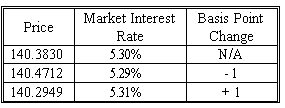### CFA Practice Question

There are 764 practice questions for this topic.

### CFA Practice Question

Cindy Bolan has an 11.00%, 9.00 bond that is selling for a price of \$140.3830 and is currently yielding 5.30%. The table below shows how the price will vary given a one basis point change in market interest rates with a modified duration of 6.28. In this instance, what is the Price Value of a Basis Point (PVBP)?A. \$0.0882
B. \$0.0705
C. \$0.1146
Explanation: The formula for the Price Value of a Basis Point (PVBP) is equal to:
modified duration * (.0001) * 100 * price = 0.0628% * the initial price of 140.3830

Another approach is to use PV- and PV+: PVBP = (140.4712 - 140.2949)/2 = 0.0882